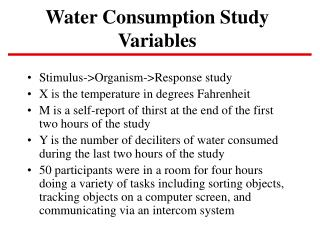# Water Consumption Study Variables - PowerPoint PPT PresentationDownload PresentationWater Consumption Study Variables

Water Consumption Study Variables
Download Presentation## Water Consumption Study Variables

- - - - - - - - - - - - - - - - - - - - - - - - - - - E N D - - - - - - - - - - - - - - - - - - - - - - - - - - -
##### Presentation Transcript

1. Water Consumption Study Variables • Stimulus->Organism->Response study • X is the temperature in degrees Fahrenheit • M is a self-report of thirst at the end of the first two hours of the study • Y is the number of deciliters of water consumed during the last two hours of the study • 50 participants were in a room for four hours doing a variety of tasks including sorting objects, tracking objects on a computer screen, and communicating via an intercom system

2. Water Consumption Study Purpose • The purpose of the study was to investigate whether persons can judge their water needs. Temperature should affect self-reported thirst which then should affect water consumption. • The accuracy of self-reported thirst is important because persons in self-contained environments need to monitor their own hydration. • The mediated effect of temperature on water consumption through self-reported thirst estimates the extent to which persons were capable of gauging their own need for water.

3. SAS Program proc reg; model y=x; model y=x m; model m=x; See handout for output.

4. SPSS Program regression /variables x y m /dependent=y /enter=x. regression /variables x y m /dependent=y /enter=x m. regression /variables x y m /dependent=m /enter x. See handout for output

5. Estimates of a, b, c, and c’ (1) Temperature (X) was significantly related to water consumption (Y) (c=.3604, sc=.1343, tc = 2.683). (2) Temperature was significantly related to self-reported thirst (M) (a=.3386, sa=.1224, ta=2.767). (3) Self-reported thirst was significantly related to water consumption controlling for temperature (b=.4510, sb=.1460, tb=3.090). -The adjusted effect of temperature was not statistically significant, (c’=.2076, sc’=.1333, tc’=1.558) and there was a drop to c’ = .2076 from c=.3604.

6. Mediation Models for Water Consumption Data Y = i1 + c X + e1 Y = -22.0505 + .3604 X + e1 (.1343) Y = i2 + c’ X + b M + e2 Y = -12.7129 + .2076 X + .4510 M + e2 (.1333) (.1460) M = i3 + a X + e3 M = -20.7024 + .3386 X + e3 (.1224)

7. Mediated Effect Measures Mediated effect ab = (.3386) (.4510) =c-c’ =.3604-.2076 =.1527. Standard error= Standard error= = z’ = .1527 / .0741 = 2.06 ab

8. Confidence Intervals for the Mediated Effect • Confidence intervals are advocated by researchers for several reasons: effect size, range of possible values, not just null hypothesis binary significance testing. For 95% confidence intervals: • Upper Confidence Interval (UCL) = ab + z.975 sab • Lower Confidence Interval (LCL) = ab + z.025 sab • For water consumption data. • UCL = .1527 + (1.96 )(.0741) = .2979 • LCL = .1527 + (-1.96) (.0741) =.0075 • 95% Confidence Interval from .0075 to .2979. The effect is statistically significant because 0 is not in the interval.

9. Special Topic: Distribution of the Product • The mediated effect is the product of two coefficients a and b. The distribution of the product has a normal distribution only in special cases. • At low values of a and b, the distribution has excess kurtosis and skewness, e.g. when a and b are both zero, kurtosis is 6. It is not surprising that the confidence limits are inaccurate if the distribution is assumed to be normal. • One solution is to use the distribution of the product in statistical tests and confidence limits.

10. Critical Values for Distribution of the Product • Because the distribution of the product is not normal, there are different critical values for the distribution for each value of a/sa and b/sb. • The critical values are -1.96 and +1.96 for the 95% confidence interval from the normal distribution. There are different upper and lower critical values for the distribution of the product. Confidence limits and significance tests are more accurate using the critical values from the distribution of the product (MacKinnon et al. 2004).

11. PRODCLIN (distribution of the PRODuct Confidence Limits for the INdirect effect) • MacKinnon, Fritz, Williams, and Lockwood, (In Press, Behavior Research Methods) describes program to compute critical values for the distribution of the product. • Web location includes programs in SAS, SPSS, and R that access a FORTRAN program. http://www.public.asu.edu/~davidpm/ripl/Prodclin/ • Input a, sa, b, sb, correlation between a and b, and Type I error rate. Output includes the input values and normal and distribution of the product confidence limits.

12. Example Calculations using the Distribution of the Product • For example, a = .3386, sa = .1224, b= .4510, sb = .1460. Enter these values in the PRODCLIN program. • PRODCLIN returns the critical value for the 2.5% percentile, Mlower =-1.6175 and Mupper = 2.2540 the critical value for the 97.5% percentile. • Use the critical values to calculate upper and lower confidence limits. • LCL= ab + Mupper sab= .1527 +(-1.6175) (.0741) UCL= ab + Mlower sab= .1527 + (2.2540 )(.0741) • Asymmetric Confidence Limits are (.0329, .3197)

13. Effect size in the water consumption study • Correlation and partial correlation effect size measures were a =.371, b= .411, and c’ = .222, c =.361 • Standardized betas were a=.371, b=.413, c’=.208, c = .361 • Proportion mediated was ab/c =.1527/.3604 = .4181 • Ratio of indirect to direct effect ab/c’ = .1527/.2076=.7259